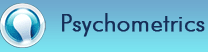• Dynamical laws are considered deterministic; implying that what works for one student will work for another with the same basic characteristics. This would support the assumption that any student with the same initial conditions, experiencing the same forces will always produce the same outcome. This has been proven false; Student development, in the most perfectly controlled conditions will rarely unfold in time in a predictable manner. This is due to the influence of a large volume of physical, mental, cognitive and emotional variables along with a myriad of external forces. Minor variations in initial conditions will lead to an astronomical number of possible outcomes. The trajectory of student analysis will diverge exponentially overtime, making a deterministic analysis model difficult to substantiate. Although student outcomes are random and often deviate from any predetermined law, appropriate pre-analysis is essential and can substantiate “a knowable outcome.” A knowable outcome is that which can be predicted based upon a thorough knowledge of all factors of influence and/or the ability to analyze outcomes of a previous event through statistical probability. The Theory of Probability is a deductive science that studies uncertain quantities relative to random events. It is the branch of mathematics that studies the likely occurrence of arbitrary outcomes in order to predict the behavior of defined systems. The basic premise is once you understand what is intended to happen, only then can you analyze the factors that support or prevent it. The study of the complexity of human performance requires a Holistic approach where an evaluation is made of the entire student rather than simply random academic outcomes. A predictive analysis is essential to enhance the analytical competence of human performance measures and the capacity for concurrent or predictive validity.